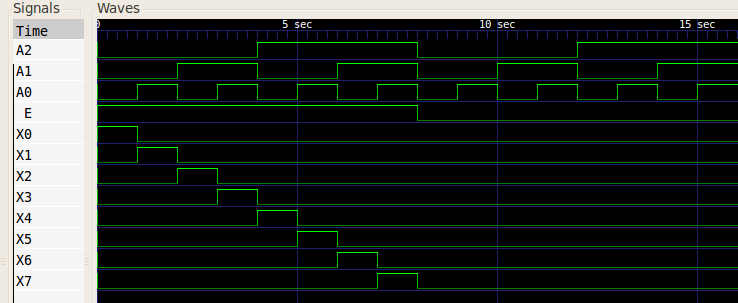# Demultiplexer

An N-line demultiplexer, a.k.a. a 1-to-N demultiplexer, is used to assign a single input line to one of N output lines. It performs the opposite job of a multiplexer. The output line which is used depends on a B-bit selection input where B=ceil(log2N). Here "ceil" is the ceiling function which returns the smallest integer greater than or equal to its argument.

The demultiplexer can be seen as a decoder which has an enable signal, and to show how, consider an 8-line demultiplexer with input line `A`, output lines `X0`, ..., `X7`, and selection bits `S0`, `S1`, and `S2`. The following table shows how to map the demultiplexer's inputs and outputs to the octal decoder's so that it performs a demultiplex function.

DemultiplexerDecoder
`Xn``Xn`
`Sn``An`
`A``E`

When simulated with test inputs, the decoder gives the following waveform which matches the specification of the demultiplexer — the binary code `A2 A1 A0` is decoded to select one of the `Xn` lines which then has the same value as the `E` input.## References

Kleitz, W. Digital Microprocessor Fundamentals. 3rd Edition. Prentice Hall, 2000.
Mano, M. Morris, and Kime, Charles R. Logic and Computer Design Fundamentals. 2nd Edition. Prentice Hall, 2000.